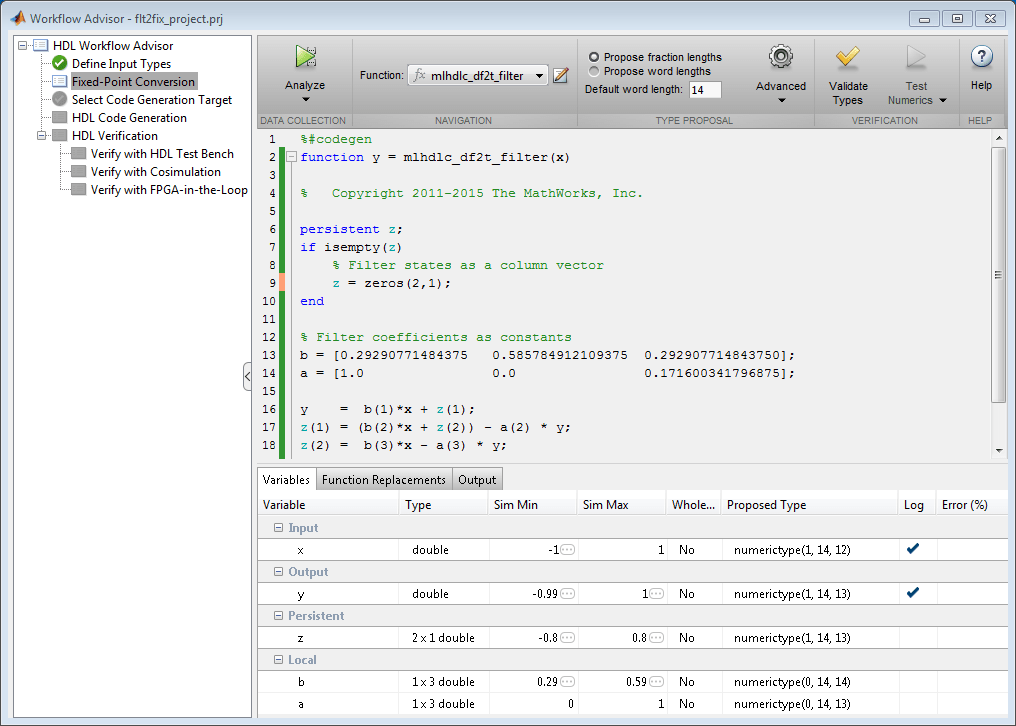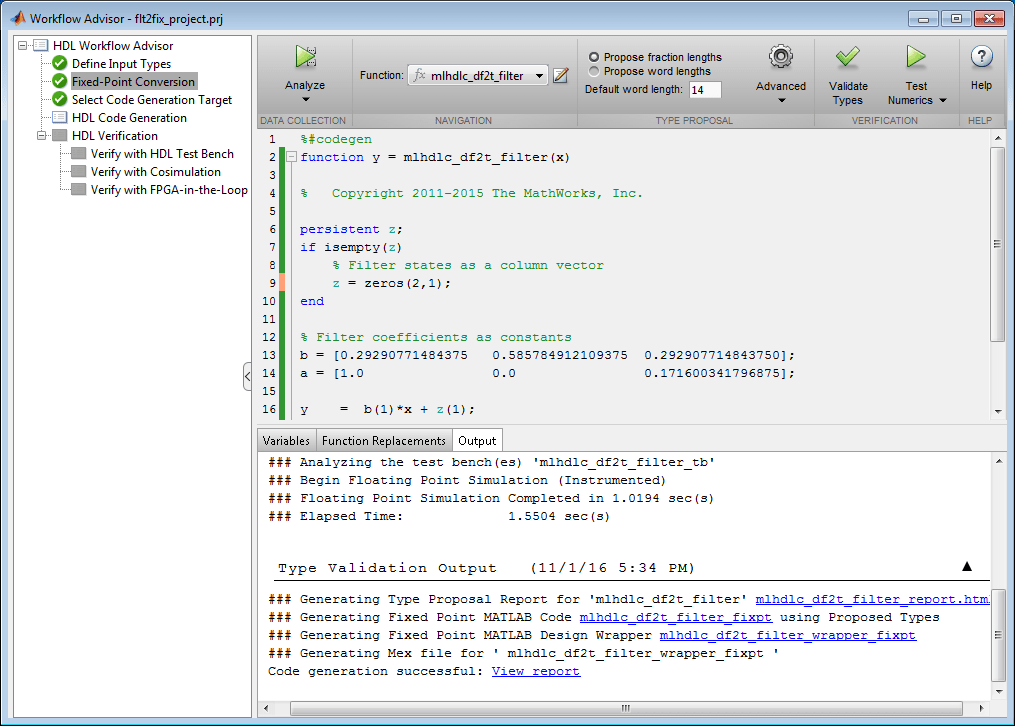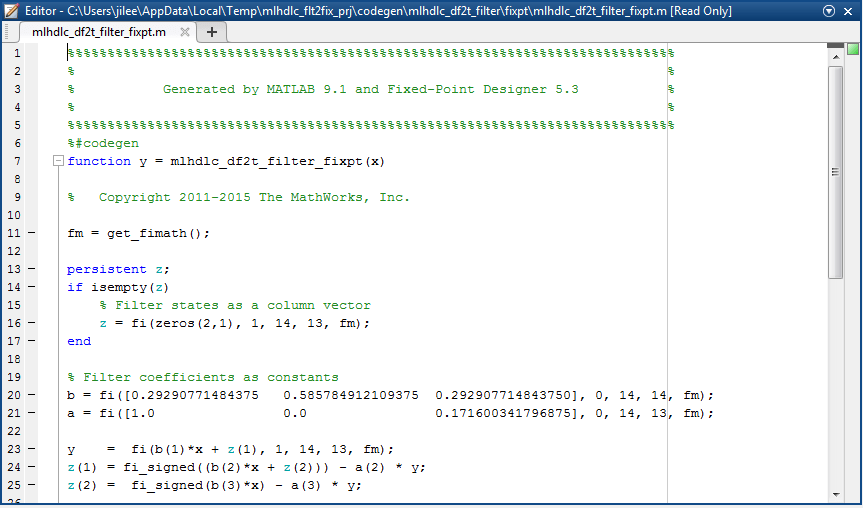# Floating-Point to Fixed-Point Conversion

Signal processing applications for reconfigurable platforms require algorithms that are typically specified using floating-point operations. However, for power, cost, and performance reasons, they are usually implemented with fixed-point operations either in software for DSP cores or as special-purpose hardware in FPGAs. Fixed-point conversion can be very challenging and time-consuming, typically demanding 25 to 50 percent of the total design and implementation time. Automated tools can simplify and accelerate the conversion process.

For software implementations, the aim is to define an optimized fixed-point specification which minimizes the code size and the execution time for a given computation accuracy constraint. This optimization is achieved through the modification of the binary point location (for scaling) and the selection of the data word length according to the different data types supported by the target processor.

For hardware implementations, the complete architecture can be optimized. An efficient implementation will minimize both the area used and the power consumption. Thus, the conversion process goal typically is focused around minimizing the operator word length.

The floating-point to fixed-point workflow is currently integrated in the HDL Workflow Advisor as described in Get Started with MATLAB to HDL Workflow.

### Introduction

The floating-point to fixed-point conversion workflow in HDL Coder™ includes the following steps:

1. Verify that the floating-point design is compatible with code generation.

2. Compute fixed-point types based on the simulation of the testbench.

3. Generate readable and traceable fixed-point MATLAB code by applying proposed types.

4. Verify the generated fixed-point design.

5. Compare the numerical accuracy of the generated fixed-point code with the original floating point code.

### MATLAB Design

The MATLAB code used in this example is a simple second-order direct-form 2 transposed filter. This example also contains a MATLAB testbench that exercises the filter.

```design_name = 'mlhdlc_df2t_filter'; testbench_name = 'mlhdlc_df2t_filter_tb'; ```

Examine the MATLAB design.

```type(design_name); ```
```%#codegen function y = mlhdlc_df2t_filter(x) % Copyright 2011-2015 The MathWorks, Inc. persistent z; if isempty(z) % Filter states as a column vector z = zeros(2,1); end % Filter coefficients as constants b = [0.29290771484375 0.585784912109375 0.292907714843750]; a = [1.0 0.0 0.171600341796875]; y = b(1)*x + z(1); z(1) = (b(2)*x + z(2)) - a(2) * y; z(2) = b(3)*x - a(3) * y; end ```

For the floating-point to fixed-point workflow, it is desirable to have a complete testbench. The quality of the proposed fixed-point data types depends on how well the testbench covers the dynamic range of the design with the desired accuracy.

For details on requirements for floating-point design and the testbench, see Floating-Point Design Structure structure section of Working with Generated Fixed-Point Files.

```type(testbench_name); ```
``` % % Copyright 2011-2015 The MathWorks, Inc. Fs = 256; % Sampling frequency Ts = 1/Fs; % Sample time t = 0:Ts:1-Ts; % Time vector from 0 to 1 second f1 = Fs/2; % Target frequency of chirp set to Nyquist in = sin(pi*f1*t.^2); % Linear chirp from 0 to Fs/2 Hz in 1 second out = zeros(size(in)); % Output the same size as the input for ii=1:length(in) out(ii) = mlhdlc_df2t_filter(in(ii)); end % Plot figure('Name', [mfilename, '_plot']); subplot(2,1,1); plot(in); xlabel('Time') ylabel('Amplitude') title('Input Signal (with Noise)') subplot(2,1,2); plot(out); xlabel('Time') ylabel('Amplitude') title('Output Signal (filtered)') ```

### Create a New Folder and Copy Relevant Files

Execute the following lines of code to copy the necessary example files into a temporary folder.

```mlhdlc_demo_dir = fullfile(matlabroot, 'toolbox', 'hdlcoder', 'hdlcoderdemos', 'matlabhdlcoderdemos'); mlhdlc_temp_dir = [tempdir 'mlhdlc_flt2fix_prj']; % create a temporary folder and copy the MATLAB files cd(tempdir); [~, ~, ~] = rmdir(mlhdlc_temp_dir, 's'); mkdir(mlhdlc_temp_dir); cd(mlhdlc_temp_dir); copyfile(fullfile(mlhdlc_demo_dir, [design_name,'.m*']), mlhdlc_temp_dir); copyfile(fullfile(mlhdlc_demo_dir, [testbench_name,'.m*']), mlhdlc_temp_dir); ```

### Simulate the Design

Simulate the design with the test bench prior to code generation to make sure there are no runtime errors.

```mlhdlc_df2t_filter_tb ```### Create a New HDL Coder Project

To create a new project, enter the following command:

```coder -hdlcoder -new flt2fix_project ```

Next, add the file 'mlhdlc_filter.m' to the project as the MATLAB Function and 'mlhdlc_filter_tb.m' as the MATLAB Test Bench.

Refer to Get Started with MATLAB to HDL Workflow for a more complete tutorial on creating and populating MATLAB HDL Coder projects.

### Fixed-Point Code Generation Workflow

The floating-point to fixed-point conversion workflow allows you to:

• Verify that the floating-point design is code generation compliant

• Propose fixed-point types based on simulation data and word length settings

• Allow the user to manually adjust the proposed fixed-point types

• Validate the proposed fixed-point types

• Verify that the generated fixed-point MATLAB code has the desired numeric accuracy

### Step 1: Launch Workflow Advisor

1. Click on the Workflow Advisor button to launch the HDL Workflow Advisor.

2. Choose Convert to fixed-point at build time for the option Fixed-point conversion.### Step 2: Define Input Types

In this step you can define input types manually or by specifying and running the testbench.

1. Click Run to execute this step.

After simulation notice that the input variable `x` is defined as scalar double, `double(1x1)`.

### Step 3: Run Simulation

1. Click on the Fixed-Point Conversion step.

The design is compiled with the input types defined in the previous step and after the compilation is successful the variable table shows inferred types for all the functions in the design.

In this step, the original design is instrumented so that the minimum and maximum values for all variables in the design are collected during simulation.1. Click on the 'Analyze' button.

Notice that the 'Sim Min' and 'Sim Max' table is now populated with simulation ranges. Fixed-point types are proposed based on the default word length settings.At this stage, based on computed simulation ranges for all variables, you can compute:

• Fraction lengths for a given fixed word length setting, or

• Word lengths for a given fixed fraction length setting.

The type table contains the following information for each variable existing in the floating-point MATLAB design, organized by function:

• Sim Min: The minimum value assigned to the variable during simulation.

• Sim Max: The maximum value assigned to the variable during simulation.

• Whole Number: Whether all values assigned during simulation are integers.

The type proposal step uses the above information and combines it with the user-specified word length settings to propose a fixed-point type for each variable.

You can also enable the Log histogram data option in the Analyze button drop-down menu to enable logging of histogram data.The histogram view concisely gives information about dynamic range of the simulation data for a variable. The x-axis correspond to bit weights and y-axis represents number of occurrences. The proposed numeric type information is overlaid on top of this graph and is editable. Moving the bounding white box left or right changes the position of binary point. Moving the right or left edges correspondingly change fraction length or wordlength. All the changes made to the proposed type are saved in the project.

### Step 4: Validate types

In this step, the fixed-point types from the previous step are used to generate a fixed-point MATLAB design from the original floating-point implementation.

1. Click on the Validate Types button.The generated code and other conversion artifacts are available via hyperlinks in the output window. The fixed-point types are explicitly shown in the generated MATLAB code.### Step 5: Test Numerics

1. Click on the Test Numerics button.

In this step, the generated fixed-point code is executed using MATLAB Coder.

If you enable the Log all inputs and outputs for comparison plots option on the Test Numerics pane, an additional plot is generated for each scalar output that shows the floating point and fixed point results, as well as the difference between the two. For non-scalar outputs, only the error information is shown.### Step 6: Iterate on the Results

If the numerical results do not meet your desired accuracy after fixed-point simulation, you can return to the Propose Fixed-Point Types step in the Workflow Advisor. Adjust the word length settings or individually modify types as desired, and repeat the rest of the steps in the workflow until you achieve your desired results.

Refer to Fixed-Point Type Conversion and Refinement for more details on how to iterate and refine the numerics of the algorithm in the generated fixed-point code.

### Clean up the Generated Files

Run the following commands to clean up the temporary project folder.

```mlhdlc_demo_dir = fullfile(matlabroot, 'toolbox', 'hdlcoder', 'hdlcoderdemos', 'matlabhdlcoderdemos'); mlhdlc_temp_dir = [tempdir 'mlhdlc_flt2fix_prj']; clear mex; cd (mlhdlc_demo_dir); rmdir(mlhdlc_temp_dir, 's'); ```